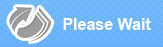Close

# TI-84 Plus Graphing Calculator

• Binding: Hardware Or Equipment
• Publisher: School Supplies
• ISBN: 0033317192120
new \$156.00
FREE economy shipping!
Description: Advanced functions accessed through pull-down display menus. Real and complex numbers calculated to 14-digit accuracy and displayed with 10 digits plus a 2-digit exponent. Graphs 10 rectangular functions, 6 parametric expressions, 6 polar expressions and 3 recursively-defined sequences. Up to 10 graphing functions defined, saved, graphed and analyzed at one time. Sequence graphing mode shows time series plot, and phase plots. Function evaluation table shows numeric evaluation of functions in table format. Interactive analysis of function values, roots, maximums, minimums, integrals and derivatives. 7 different graph styles for differentiating the look of each graph drawn. Matrix operations including inverse, determinant, transpose, augment, reduced row echelon form and elementary row operations. List-based one- and two-variable statistical analysis, including logistic, sinusoidal, median-median, linear, logarithmic, exponential, power, and quadratic polynomial regression models.
Expand description